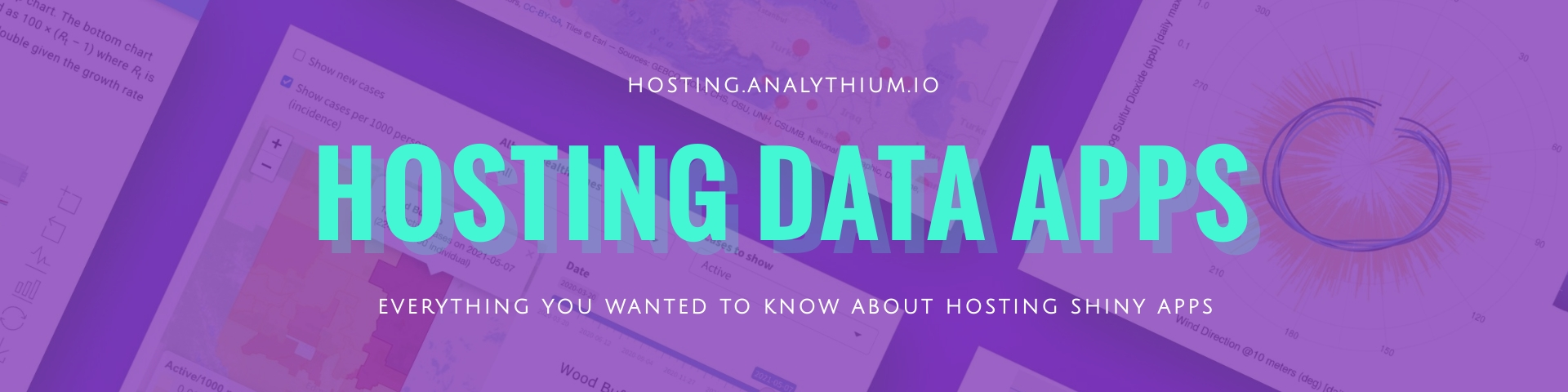# Shiny UIThe user interface (UI) object controls the layout and appearance of the Shiny app.

library(shiny)

The UI is defined as:

ui <- fluidPage(
mainPanel(
sliderInput("obs",
"Number of observations",
min = 1,
max = 5000,
value = 100),
plotOutput("distPlot")
)
)

The `fluidPage()` function renders the main Shiny interface, a range slider input is nested inside alongside the plot output. The slider with ID `"obs"` controls the number of observations (range between 1 and 5000, value set to 100). The plot with ID `"distPlot"` will show the distribution of the random numbers (we'll see this later).

If we print the `ui` object, we get the following HTML output:

<div class="container-fluid">
<div class="col-sm-8">
<div class="form-group shiny-input-container">
<label class="control-label" for="obs">
Number of observations
</label>
<input class="js-range-slider" id="obs" data-min="1"
data-max="5000" data-from="100" data-step="1"
data-grid="true" data-grid-num="9.998"
data-grid-snap="false" data-prettify-separator=","
data-prettify-enabled="true" data-keyboard="true"
data-data-type="number"
/>
</div>
<div id="distPlot" class="shiny-plot-output"
style="width: 100% ; height: 400px"
>
</div>
</div>
</div>

You can see here the fluid container, a column, and inside the single column layout the range slider and the plot.

This is going to be added to the body of the HTML page rendered by Shiny. The final HTML page will also contain all the JavaScript and CSS dependencies required to make the app interactive and styled properly.